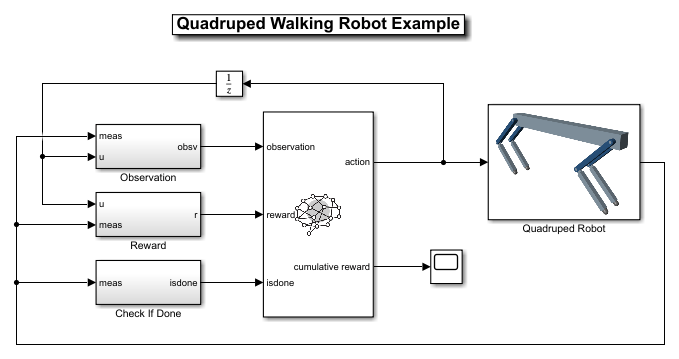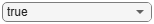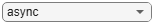# Quadruped Robot Locomotion Using DDPG Agent

This example shows how to train a quadruped robot to walk using a deep deterministic policy gradient (DDPG) agent. The robot in this example is modeled using Simscape™ Multibody™. For more information on DDPG agents, see Deep Deterministic Policy Gradient (DDPG) Agents.Load the necessary parameters into the base workspace in MATLAB®.

`initializeRobotParameters`

The environment for this example is a quadruped robot, and the training goal is to make the robot walk in a straight line using minimal control effort.

Open the model.

```mdl = "rlQuadrupedRobot"; open_system(mdl)```The robot is modeled using Simscape Multibody with its main structural components consisting of four legs and a torso. The legs are connected to the torso through revolute joints that enable rotation of the legs with respect to the torso. The joints are actuated by torque signals provided by the RL Agent.

#### Observations

The robot environment provides 44 observations to the agent, each normalized between –1 and 1. These observations are:

• Y (vertical) and Y (lateral) position of the torso center of mass

• Quaternion representing the orientation of the torso

• X (forward), Y (vertical), and Z (lateral) velocities of the torso at the center of mass

• Roll, pitch, and yaw rates of the torso

• Angular positions and velocities of the hip and knee joints for each leg

• Normal and friction force due to ground contact for each leg

• Action values (torque for each joint) from the previous time step

For all four legs, the initial values for the hip and knee joint angles are set to –0.8234 and 1.6468 radians, respectively. The neutral positions of the joints are set at 0 radian. The legs are in neutral position when they are stretched to their maximum and are aligned perpendicularly to the ground.

#### Actions

The agent generates eight actions normalized between –1 and 1. After multiplying with a scaling factor, these correspond to the eight joint torque signals for the revolute joints. The overall joint torque bounds are +/– 10 N·m for each joint.

#### Reward

The following reward is provided to the agent at each time step during training. This reward function encourages the agent to move forward by providing a positive reward for positive forward velocity. It also encourages the agent to avoid early termination by providing a constant reward ($25{T}_{s}/{T}_{f}$) at each time step. The remaining terms in the reward function are penalties that discourage unwanted states, such as large deviations from the desired height and orientation or the use of excessive joint torques.

`${r}_{t}={v}_{x}+25\frac{{T}_{s}}{{T}_{f}}-50{\underset{}{\overset{ˆ}{y}}}^{2}-20{\theta }^{2}-0.02\sum _{i}{{u}_{t-1}^{i}}^{2}$`

where

• ${v}_{x}$ is the velocity of the torso's center of mass in the x-direction.

• ${T}_{s}$ and ${T}_{f}$ are the sample time and final simulation time of the environment, respectively.

• $\underset{}{\overset{ˆ}{y}}$ is the scaled height error of the torso's center of mass from the desired height of 0.75 m.

• $\theta$ is the pitch angle of the torso.

• ${u}_{t-1}^{i}$is the action value for joint $\mathit{i}$ from the previous time step.

#### Episode Termination

During training or simulation, the episode terminates if any of the following situations occur.

• The height of the torso center of mass from the ground is below 0.5 m (fallen).

• The head or tail of the torso is below the ground.

• Any knee joint is below the ground.

• Roll, pitch, or yaw angles are outside bounds (+/– 0.1745, +/– 0.1745, and +/– 0.3491 radians, respectively).

### Create Environment Interface

Specify the parameters for the observation set.

```numObs = 44; obsInfo = rlNumericSpec([numObs 1]); obsInfo.Name = "observations";```

Specify the parameters for the action set.

```numAct = 8; actInfo = rlNumericSpec([numAct 1],LowerLimit=-1,UpperLimit= 1); actInfo.Name = "torque";```

Create the environment using the reinforcement learning model.

```blk = mdl + "/RL Agent"; env = rlSimulinkEnv(mdl,blk,obsInfo,actInfo);```

During training, the reset function introduces random deviations into the initial joint angles and angular velocities.

`env.ResetFcn = @quadrupedResetFcn;`

### Create DDPG agent

The DDPG agent approximates the long-term reward given observations and actions by using a critic value function representation. The agent also decides which action to take given the observations, using an actor representation. The actor and critic networks for this example are inspired by .

For more information on creating a deep neural network value function representation, see Create Policies and Value Functions. For an example that creates neural networks for DDPG agents, see Compare DDPG Agent to LQR Controller.

Set the random seed.

`rng(0)`

Create the networks in the MATLAB workspace using the `createNetworks` helper function.

`createNetworks`

You can also create your actor and critic networks interactively using the Deep Network Designer app.

View the critic network configuration.

`plot(criticNetwork)`View the actor network configuration.

`plot(actorNetwork)`Specify the agent options using `rlDDPGAgentOptions`.

```agentOptions = rlDDPGAgentOptions(); agentOptions.SampleTime = Ts; agentOptions.DiscountFactor = 0.99; agentOptions.MiniBatchSize = 128; agentOptions.ExperienceBufferLength = 1e6; agentOptions.TargetSmoothFactor = 1e-3; agentOptions.NoiseOptions.MeanAttractionConstant = 0.15; agentOptions.NoiseOptions.Variance = 0.1;```

Specify optimizer options for the actor and critic.

```agentOptions.ActorOptimizerOptions.Algorithm = "adam"; agentOptions.ActorOptimizerOptions.LearnRate = 1e-4; agentOptions.ActorOptimizerOptions.GradientThreshold = 1; agentOptions.ActorOptimizerOptions.L2RegularizationFactor = 1e-5; agentOptions.CriticOptimizerOptions.Algorithm = "adam"; agentOptions.CriticOptimizerOptions.LearnRate = 1e-3; agentOptions.CriticOptimizerOptions.GradientThreshold = 1; agentOptions.CriticOptimizerOptions.L2RegularizationFactor = 2e-4;```

Create the `rlDDPGAgent` object for the agent.

`agent = rlDDPGAgent(actor,critic,agentOptions);`

### Specify Training Options

To train the agent, first specify the following training options:

• Run each training episode for at most 10,000 episodes, with each episode lasting at most `maxSteps` time steps.

• Display the training progress in the Episode Manager dialog box (set the `Plots` option).

• Stop training when the agent receives an average cumulative reward greater than 190 over 250 consecutive episodes.

```trainOpts = rlTrainingOptions(... MaxEpisodes=10000,... MaxStepsPerEpisode=floor(Tf/Ts),... ScoreAveragingWindowLength=250,... Plots="training-progress",... StopTrainingCriteria="AverageReward",... StopTrainingValue=210);```

To train the agent in parallel, specify the following training options. Training in parallel requires Parallel Computing Toolbox™ software. If you do not have Parallel Computing Toolbox™ software installed, set `UseParallel` to `false`.

• Set the `UseParallel` option to t`rue`.

• Train the agent in parallel asynchronously.

```trainOpts.UseParallel = true; trainOpts.ParallelizationOptions.Mode = "async";```

### Train Agent

Train the agent using the `train` function. Due to the complexity of the robot model, this process is computationally intensive and takes several hours to complete. To save time while running this example, load a pretrained agent by setting `doTraining` to `false`. To train the agent yourself, set `doTraining` to `true`. Due to the randomness of parallel training, you can expect different training results from the plot below.

```doTraining = false; if doTraining % Train the agent. trainingStats = train(agent,env,trainOpts); else % Load pretrained agent parameters for the example. load("rlQuadrupedAgentParams.mat","params") setLearnableParameters(agent, params); end```### Simulate Trained Agent

Fix the random generator seed for reproducibility.

`rng(0)`

To validate the performance of the trained agent, simulate it within the robot environment. For more information on agent simulation, see `rlSimulationOptions` and `sim`.

```simOptions = rlSimulationOptions(MaxSteps=floor(Tf/Ts)); experience = sim(env,agent,simOptions);```### References

 Heess, Nicolas, Dhruva TB, Srinivasan Sriram, Jay Lemmon, Josh Merel, Greg Wayne, Yuval Tassa, et al. ‘Emergence of Locomotion Behaviours in Rich Environments’. ArXiv:1707.02286 [Cs], 10 July 2017. https://arxiv.org/abs/1707.02286.

 Lillicrap, Timothy P., Jonathan J. Hunt, Alexander Pritzel, Nicolas Heess, Tom Erez, Yuval Tassa, David Silver, and Daan Wierstra. ‘Continuous Control with Deep Reinforcement Learning’. ArXiv:1509.02971 [Cs, Stat], 5 July 2019. https://arxiv.org/abs/1509.02971.